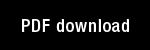A prediction theory for some nonlinear functional-differential equations, II: Learning of patterns

Author(s): Grossberg, S. |

Year: 1968

Citation: Journal of Mathematical Analysis and Applications, 22, 490-522

Abstract: This paper studies the following system of nonlinear difference-differentialequations: [...](3)where i, j, k = 1, 2,..., n, and /3 > 0. We will establish global limit andoscillation theorems for the nonnegative solutions of (*) when (*) has any fixed number of variables (n >, 2) and r is any fixed nonnegative time lag.(*) arises as an example of a nonstationary prediction theory, or learningtheory, whose goal is to discuss the prediction of individual events, in a fixed order, and at prescribed times (,). In this theory, (*) describesa machine Al subjected to inputs C = (I1 , I_ ,..., I,,) by an experimenter F,,who records the outputs X = (xl , x, ,..., xn) created thereby. I; has onlythe inputs C and outputs X at his disposal with which to describe ('*), andin terns of these variables (*) takes the formX(t) =-- --X(t) + I3(X,) X(t - r) -1- C(t), (4)where 11(.X) is a matrix of nonlinear functionals of X(zv) evaluated at allpast times zv e [ --r, t] with. entries
The machine Al therefore obeys the functional-differential equations (4)-(5),and 13(.x",) contains the "memory" of ill. Our global limit and oscillationtheorems for (*) can be interpreted as learning experiments performed by Eon !ll to study how Al learns, remembers what it has learned, and reactsto test inputs in recall experiments. In particular, (*) can learn a spatialpattern in "black and white" of arbitrary size and complexity (see ).The prediction theory in [I] introduces infinitely many nonlinear systems.Each system is characterized by an n x n "coefficient" matrix P -_ 11 p;f 11which is sernistochastic; that is, p;; > 0 and = 0 or 1. (*) ischaracterized by the stochastic matrix with entries p,; = (I/n). This matrixcan be realized as a probabilistic network G , and (*) can be interpretedas a cross-correlated flow over G  in the following way.G consists of n vertices V = {v; : i = I, 2,..., n} and n2 directed edgesE = {e; , : j, k k= 1, 2,..., n}, where ejk has v, as its initial vertex and vk asits terminal vertex. The coefficient matrix P assigns the weight Pik = (11n)to e;k . Since every vertex v; is connected to every other vertex of with equalweight, the graph G is complete. Since v; is also connected to itself, G is acomplete graph with loops. We illustrate this graph in the case n=3 in Fig.1.

Topics: Mathematical Foundations of Neural Networks,